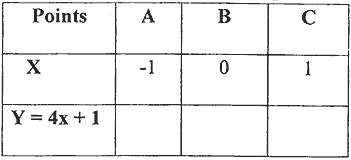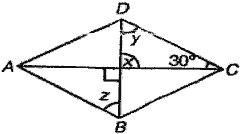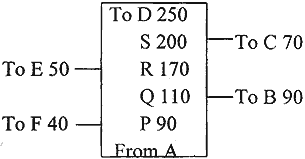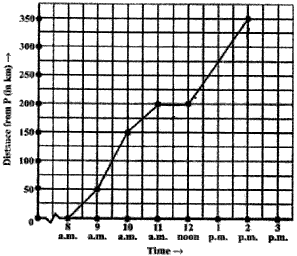Tuesday , September 27 2022# 8th Mathematics Annual Exam 2018-19

## 8th Mathematics Annual Exam (2018-19)

School Name: Venkateshwar Global School, Sector 13, Rohini, Delhi 110085 India
Time: 3 hour
M.M. 80 marks
Date: 05/02/2019
Subject: Mathematics
Class: VIII

## General Instructions:

1. All questions are compulsory.
2. Do you work neatly.
3. Do not scribble on the question paper.
4. Write your name and section on the question paper.

## Section B [2×10 = 20]

#### Question: 16. In the figure given below, PQRS is a Rhombus, state the length of PQ and ∠Q. Give reasons.## Section C [3×10 = 30]

#### Question: 22. Complete the table of values and make a graph to represent these points.## Section D [4×6 = 24]

#### Question: 27. ABCD is a Rhombus. Find the values of x,y,z and also find AD if CB = 7 cm. Give reasons for your answer. (Refer to figure given)#### Question: 29. Find the area of the following field. The measurements are in metres.#### Question: 32. The given graph describes the distances of a car from a city P at different times when it is travelling from City P to City Q, which are 350 km apart. Study the graph and answer the following:

1. How far did the car go in the first hour?
2. How much time did the car took to travel between 200 km to 350 km?
3. Did the car stop for some duration at any place? Justify your answer.
4. How much time will the whole journey take to reach city Q from P without stopping anywhere?## 11th Class English Term 2 Question Paper 2021-22

School Name: Himalaya Public School, Sector 13, Rohini, Delhi 110085 India Class: 11th Standard (CBSE) Subject: English Time …